# Terminal Value Calculation: Growth in Perpetuity & Exit Multiple Approach

Terminal Value Formula and Definition Explained with Step-by-Step Calculation of the Perpetuity Growth & Exit Multiple Method in Excel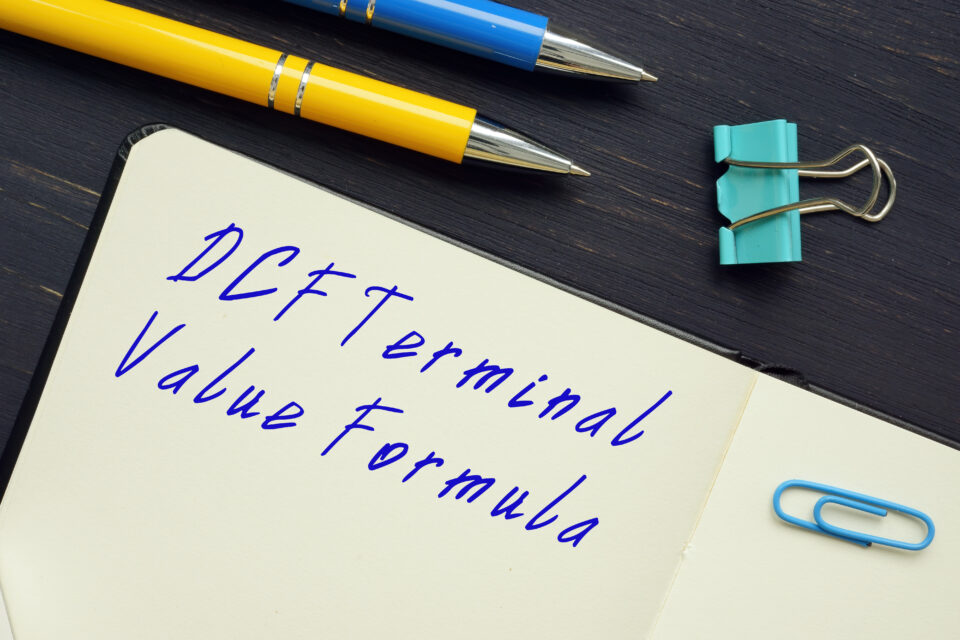Key Takeaways
• In the context of valuation, what is the conceptual meaning of the terminal value (TV)?
• What are the main steps to computing the perpetuity and exit multiple terminal value calculation?
• Name some common indicators that a company has reached a sustainable, “stable” state.
• How can the implied growth rate and exit multiple be estimated to use as a sanity check?

## Calculating the Terminal Value

Just as a brief review, the premise of the DCF approach states that an asset (the company) is worth the sum of all of its future free cash flows (FCFs) – which are discounted to the present day to account for the “time value of money” (i.e., \$1 received today is worth more than \$1 received on a later date). Since it is not feasible to project a company’s FCF indefinitely, the standard DCF model is made up of two “parts”: a FCF forecast (typically 5-10 years) and the terminal value.

The accuracy of cash flow forecasts tends to become less reliable the further into the future one goes. And eventually, simplified high-level assumptions become necessary to capture the lump sum value at the end of the forecast period.

In practice, there are two widely used methods for calculating the terminal value:

1. Growth in Perpetuity Approach
2. Exit Multiple Approach

## Growth in Perpetuity Formula

The growth in perpetuity approach attaches a constant growth rate onto the forecasted cash flows of a company after the explicit forecast period. Here, the terminal value is calculated by treating a company’s terminal year FCF as a growing perpetuity at a fixed rate.

One of the first steps to building a DCF is projecting the company’s future FCFs until its financial performance has reached a normalized “steady state”, which subsequently serves as the basis of the terminal value under the growth in perpetuity approach.

The long-term growth rate should theoretically be the growth rate that the company can sustain into perpetuity. Often, GDP growth or the risk-free rate can serve as proxies for the growth rate. Given how the terminal value accounts for a substantial portion of a company’s valuation, the terminal year must not be distorted by cyclicality or seasonality patterns.

The long-term growth rate assumption should generally range between 2% to 4% to reflect a realistic, sustainable rate. Otherwise, the implicit assumption of an excessively high growth rate (i.e., >5%) is that the company is on track to someday outpace the growth of the global economy – which is clearly an unrealistic scenario.

Closely tied to the revenue growth, the reinvestment needs of the company must have also normalized near this time, which can be signified by depreciation largely equaling capex and capex as a percentage of revenue ranging near the lower end of what is typical in the company’s industry (i.e., the majority of capex that remains is maintenance-related).

A frequent error in the terminal value calculation is cutting off the explicit forecast period too soon when the company’s cash flows have yet to reach maturity.

Taking a step back, a perpetuity is defined as security (e.g., bond) with no fixed maturity date, and the formula for calculating the present value (PV) of a perpetuity is equal to the cash flow value divided by the discount rate (i.e., expected rate of return based on the risks associated with receiving the cash flows).

For instance, if the cash flow at the end of the initial forecast period is \$100 and the discount rate is 10.0%, the terminal value comes out to \$1,000 (\$100 ÷ 10.0%).

But as mentioned earlier, the perpetuity growth method assumes that a company’s cash flows grow at a constant rate perpetually. Because of this distinction, the perpetuity formula must account for the fact that there is going to be growth in the cash flows, as well. Hence, the denominator deducts the growth rate from the discount rate.

Returning to the example from earlier, if the cash flow at the end of the initial projection period is \$100 and the discount rate is 10.0% but this time around, there is a perpetuity growth rate of 3%, the terminal value comes out as ~\$1,471, which can be calculated as ([\$100 x (1 + 3.0%)] ÷ [10.0% – 3.0%]).

The formula for calculating the terminal value under the perpetuity approach involves taking the final year FCF and growing it by the long-term growth rate assumption and then dividing that amount by the discount rate minus the perpetuity growth rate.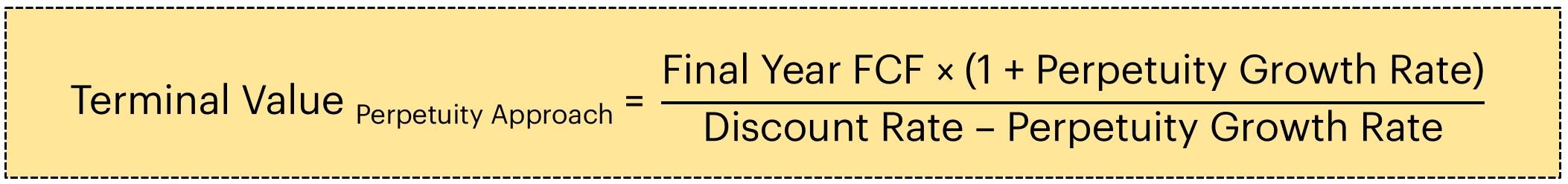Here, the terminal value is reliant on two major assumptions:

1. Discount Rate (r)
2. Perpetuity Growth Rate (g)

If the cash flows being projected are unlevered free cash flows, then the proper discount rate to use would be the weighted average cost of capital (WACC) and the ending output is going to be the enterprise value. But if the cash flows are levered FCFs, the discount rate should be the cost of equity and the equity value is the resulting output.

## Exit Multiple Approach Formula

The exit multiple approach applies a valuation multiple to a metric of the company to estimate its terminal value. In theory, the exit multiple serves as a useful point of reference for the future valuation of the target company in its mature state.

The formula for the terminal value using the exit multiple approach multiplies the value of a certain financial metric (e.g., EBITDA) in the final year of the explicit forecast period by an exit multiple assumption. The exit multiple assumption is typically derived from a peer comparables set, including through an average of current public trading multiples and multiples as derived from precedent transactions of comparable targets.The exit multiple method also comes with its share of criticism as its inclusion brings an element of relative valuation into the intrinsic valuation. The DCF model utilizes a fundamentals-oriented perspective and one of the touted benefits is its independence from prevailing market valuations, which can be prone to mispricing at times due to irrational investor sentiment (e.g., short-term overreactions).

But compared to the perpetuity growth approach, the exit multiple approach tends to be viewed more favorably because the assumptions used to calculate the terminal value can be better explained (and are thus more defensible). The growth rate in the perpetuity approach can be seen as a less rigorous, “quick and dirty” approximation – even if the values under both methods differ marginally.

In either approach, the terminal value represents the present value of the company’s cash flows in the final year of the explicit forecast period before entering the perpetuity stage (i.e. if Year 10 cash flows is used for the terminal value calculations, than the resulting terminal value derived from the methods above represent the present value of the terminal value in Year 10). Since the DCF is based on what a company is worth as of today, it is a necessary to discount the future terminal value back to the present date (i.e. in the aforementioned example, the Year 10 terminal value needs to be discounted back to the equivalent Year 0 terminal value).## Terminal Value Sanity Checks

Typically, the perpetuity growth approach is used in conjunction with the exit multiple approach to cross-check the implied exit multiple – and vice versa, as each serves as a sanity check on the other. Unless there are atypical circumstances such as time constraints or absence of data surrounding the valuation, the calculation under both methods is normally listed out side-by-side.

For example, if the implied perpetuity growth rate based on the exit multiple approach seems excessively low or high, it may be an indication that the assumptions might require adjusting.If the exit multiple approach was used to calculate the terminal value, it’s important to cross-check the amount by backing into an implied growth rate to confirm that it’s reasonable. In effect, the terminal value under either approach should be reasonably close – albeit, the exit multiple approach is viewed more favorably in practice due to the relative ease of justifying the assumptions used, especially since the DCF method is intended to be an intrinsic, cash-flow oriented valuation.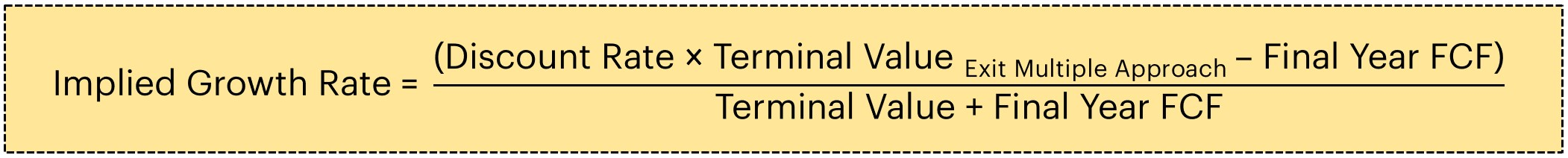So far, we’ve discussed the meaning and rationale for using the terminal value in the context of performing cash-flow-based valuations, as well as the two methods to calculate the terminal value. Now that the core concepts have been explained, we can move onto a step-by-step calculation. To follow along with the example tutorial, fill out the form below to access the spreadsheet.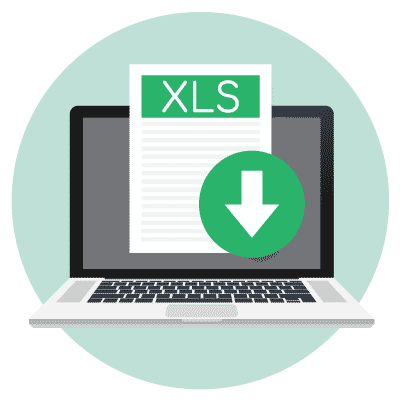Submitting ...

## Stage 1 Cash Flow Forecast

Let’s get started with the projected figures for our hypothetical company’s EBITDA and free cash flow. In the last twelve months (LTM), EBITDA was \$50mm and unlevered free cash flow was \$30mm. From Year 1 to Year 5 – the forecasted range of stage 1 cash flows – EBITDA grows by \$2mm each year and the 60% FCF to EBITDA ratio (\$30mm in FCF ÷ \$50mm in EBITDA) is assumed to remain fixed. This assumption is extrapolated for each forecasted period. For example, the FCF in “Year 5” is \$36mm, which is computed by multiplying the \$60mm in EBITDA by the 60.0% FCF conversion assumption.

In the next step, we’ll be summing up the PV of the projected cash flows over the next five years – i.e., how much all of the forecasted cash flows are worth today. Since the discount rate assumption is hardcoded as 10.0%, we can divide each free cash flow amount by (1 + the discount rate), raised to the power of the period number.

For purposes of simplicity, the mid-year convention is not used, so the cash flows are being discounted as if they are being received at the end of each period. Note the appropriate cash flows to select in the range should only consist of future cash flows and exclude any historical cash flows (e.g., Year 0).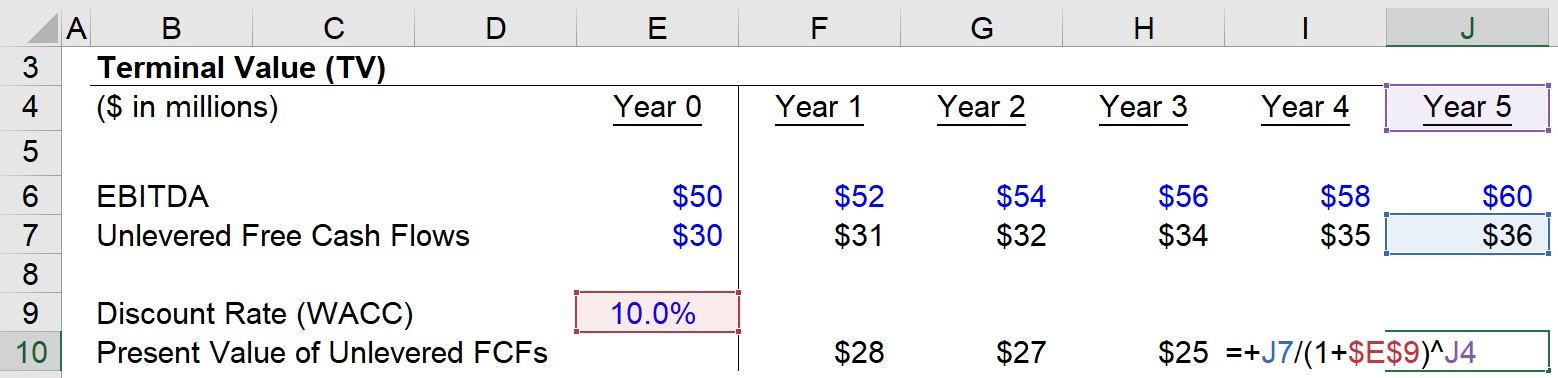Once we discount each FCF and sum up the values, we get \$127mm as the PV of the stage 1 FCFs – and this amount remains constant under either approach.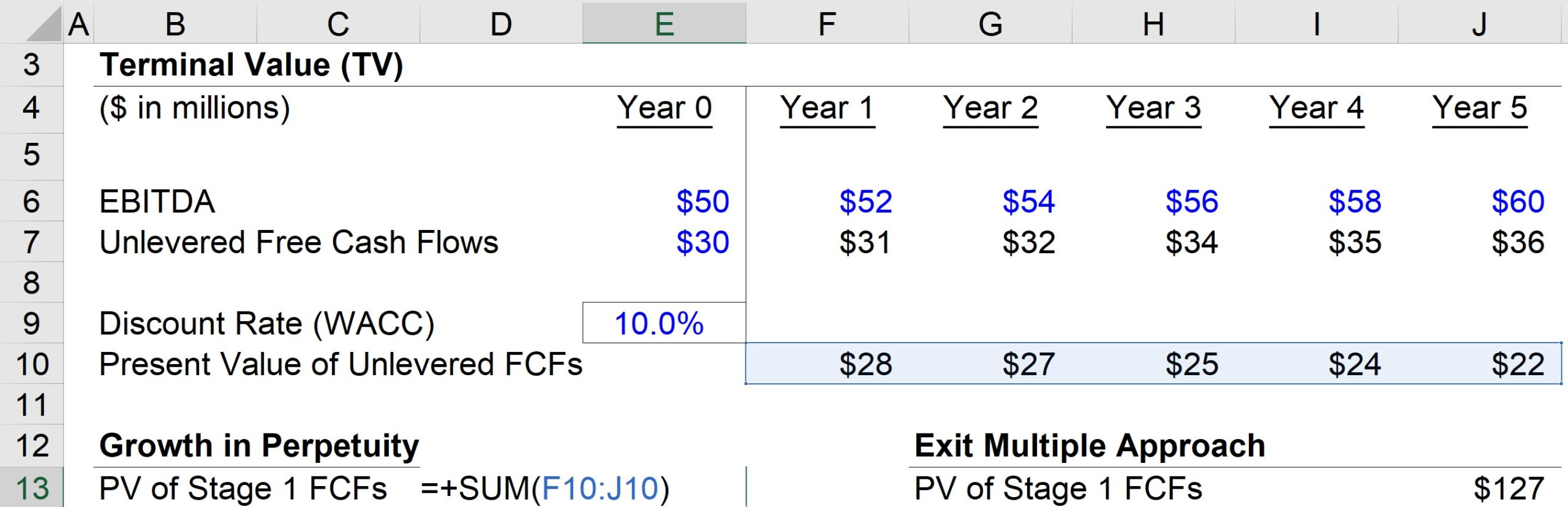## Growth in Perpetuity Approach Calculation Example

Now that we’ve finished projecting the stage 1 FCFs, we can move onto calculating the terminal value under the growth in perpetuity approach. To be conservative, we’ll be using 2.5% as the long-term growth rate assumption. Next, the Year 5 FCF of \$36mm is going to be multiplied by the 2.5% growth rate to arrive at \$37mm for the FCF value in the next year, which will then be inserted into the formula for the terminal value calculation.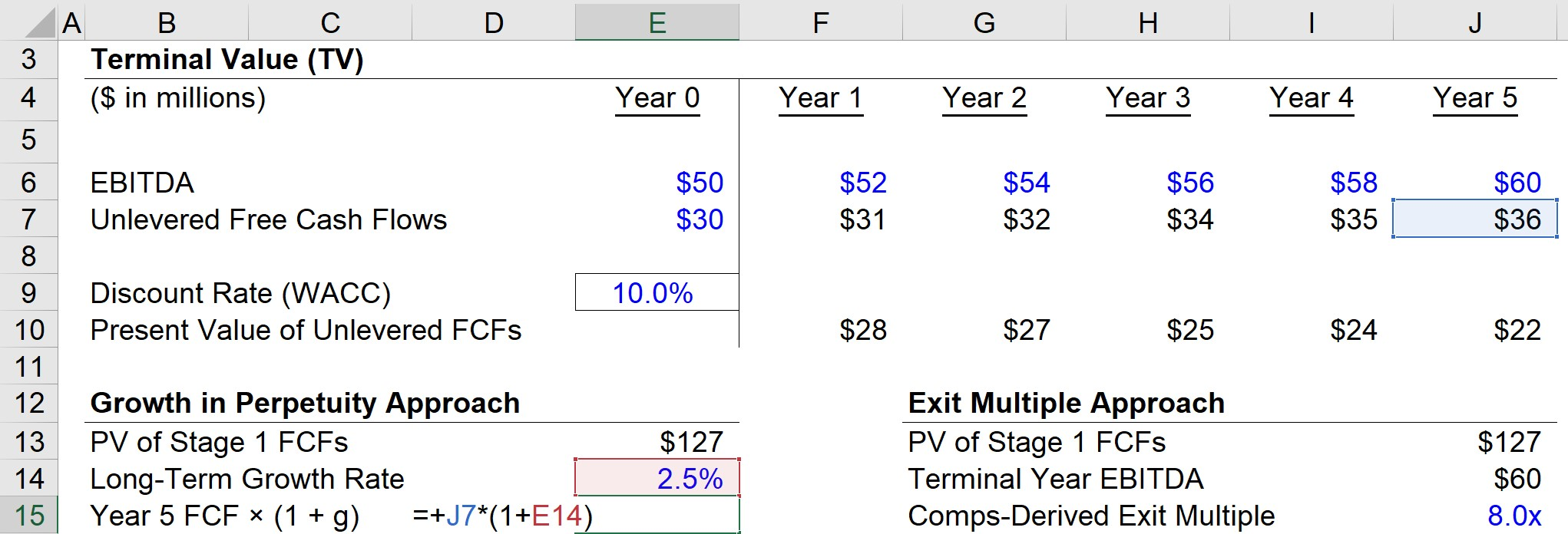Upon dividing the \$37mm by the denominator consisting of the discount rate of 10% minus the 2.5%, we get \$492mm as the terminal value in Year 5.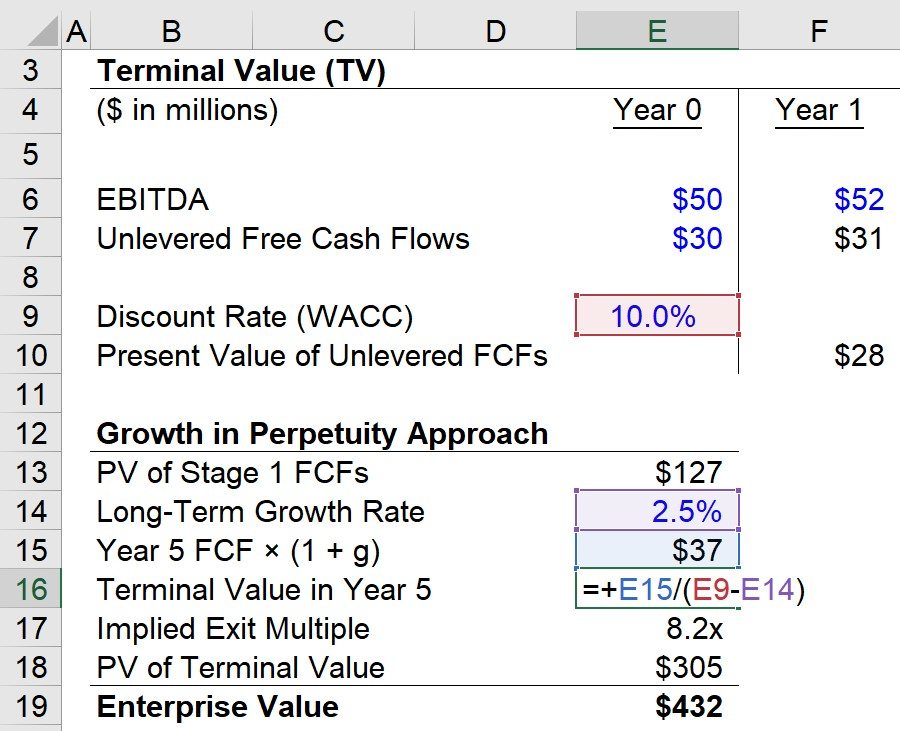But another important step is that the terminal value we calculated is as of Year 5, while the DCF valuation is based on the value in relation to today. Thus, using the formula shown below, we discount the terminal value back to the present date to get \$305mm as the PV of the terminal value.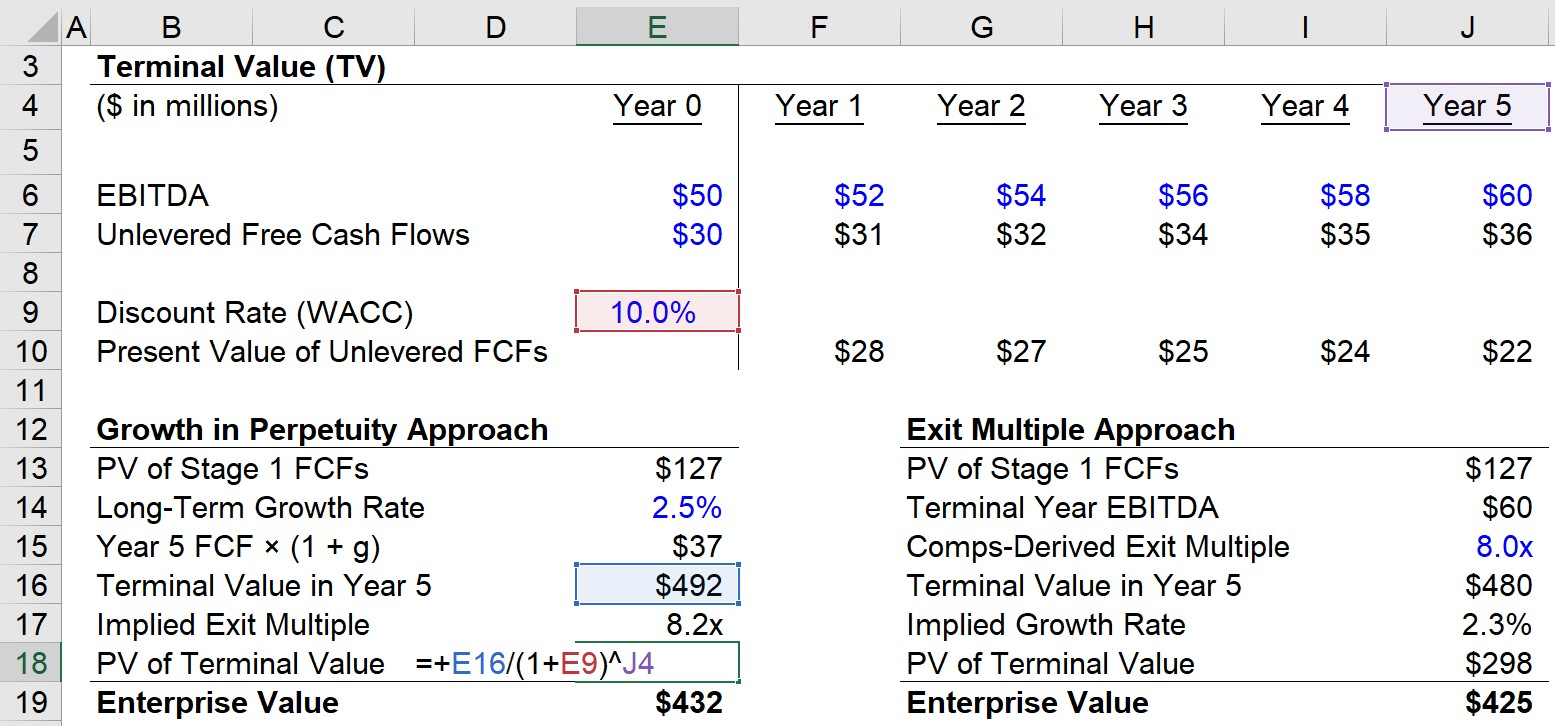If we sum up the two values – the \$127mm PV of stage 1 FCFs and \$305mm PV of the terminal value – we get \$432mm as the implied enterprise value.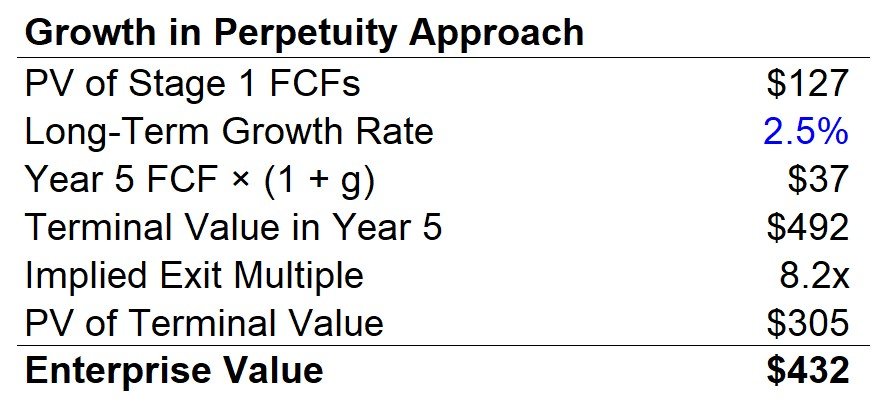## Exit Multiple Approach Calculation Example

Moving onto the other terminal value calculation method, we’ll now walk through the exit multiple approach. The \$127mm in PV of stage 1 FCFs was previously calculated and can just be linked to the matching cell on the left. Then, we’ll grab the terminal year EBITDA, which is \$60mm in Year 5.

The exit multiple assumption we’ll use here is going to be 8.0x. By multiplying the \$60mm in terminal year EBITDA by the comps-derived exit multiple assumption of 8.0x, we get \$480mm as the terminal value in Year 5. But once again, the PV of this amount must be calculated by dividing \$480mm by (1 + 10% discount rate) raised to the power of 5, which comes out to \$298mm.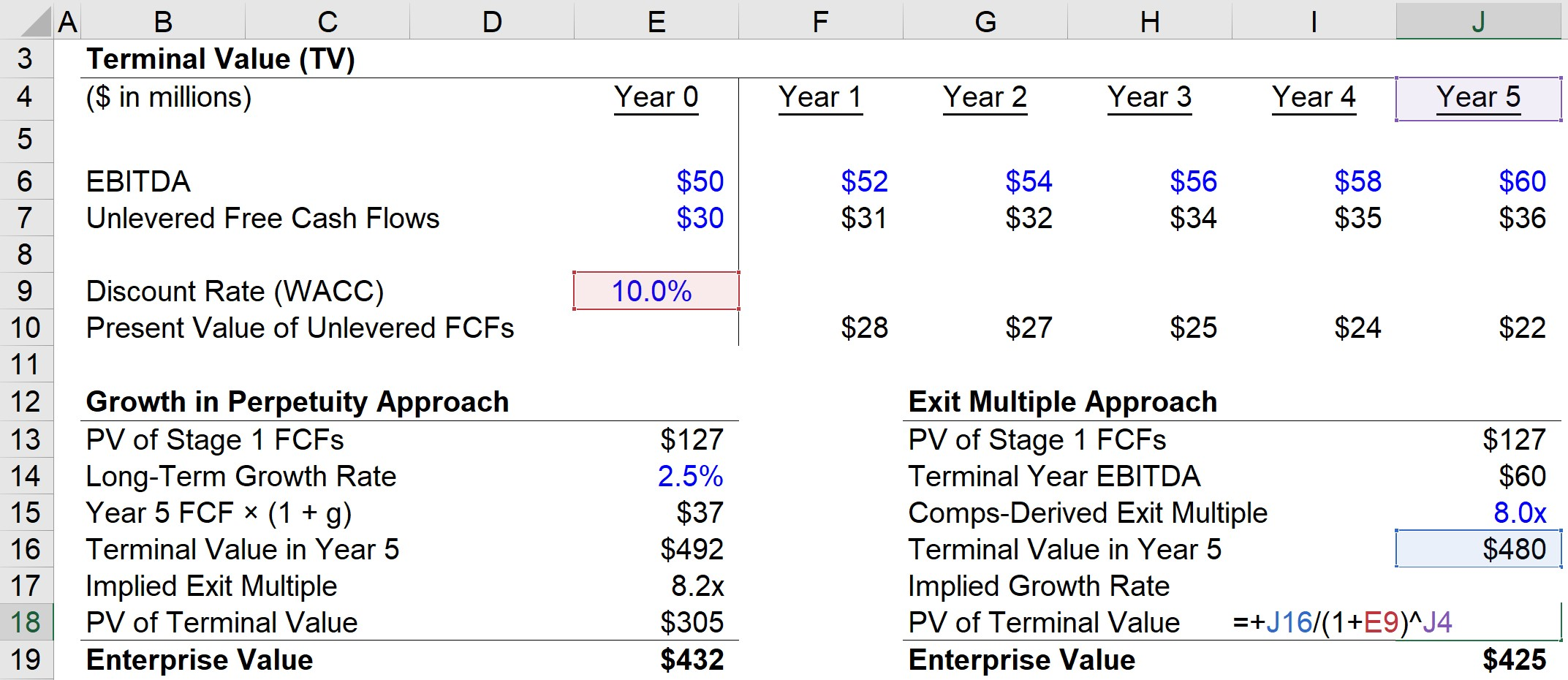The enterprise value output based on the exit multiple approach is shown below. The \$425mm TEV was calculated by taking the sum of the \$127mm PV of stage 1 FCFs and the \$298mm in the PV of the terminal value.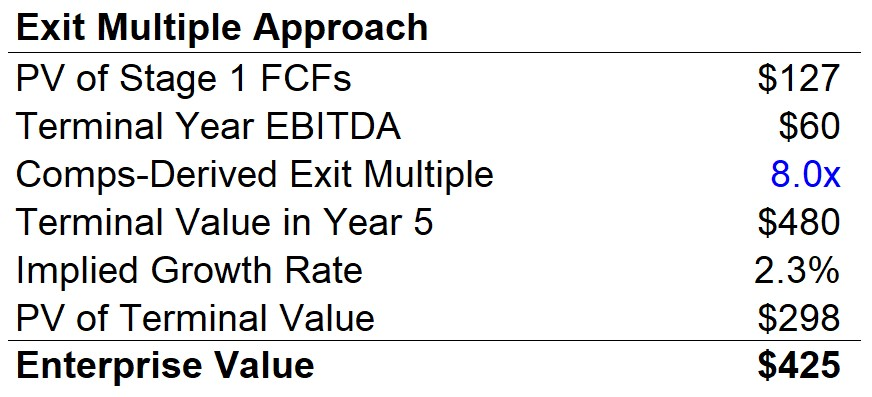## Implied Growth Rate and Exit Multiple Calculation

In our final section, we’ll perform sanity checks on our terminal values calculations to determine whether our assumptions were reasonable or not. Starting with the growth in perpetuity approach, we can back out the implied exit multiple by dividing the terminal value in Year 5 (\$492mm) by the final year EBITDA (\$60mm), which comes out to an implied exit multiple of 8.2x.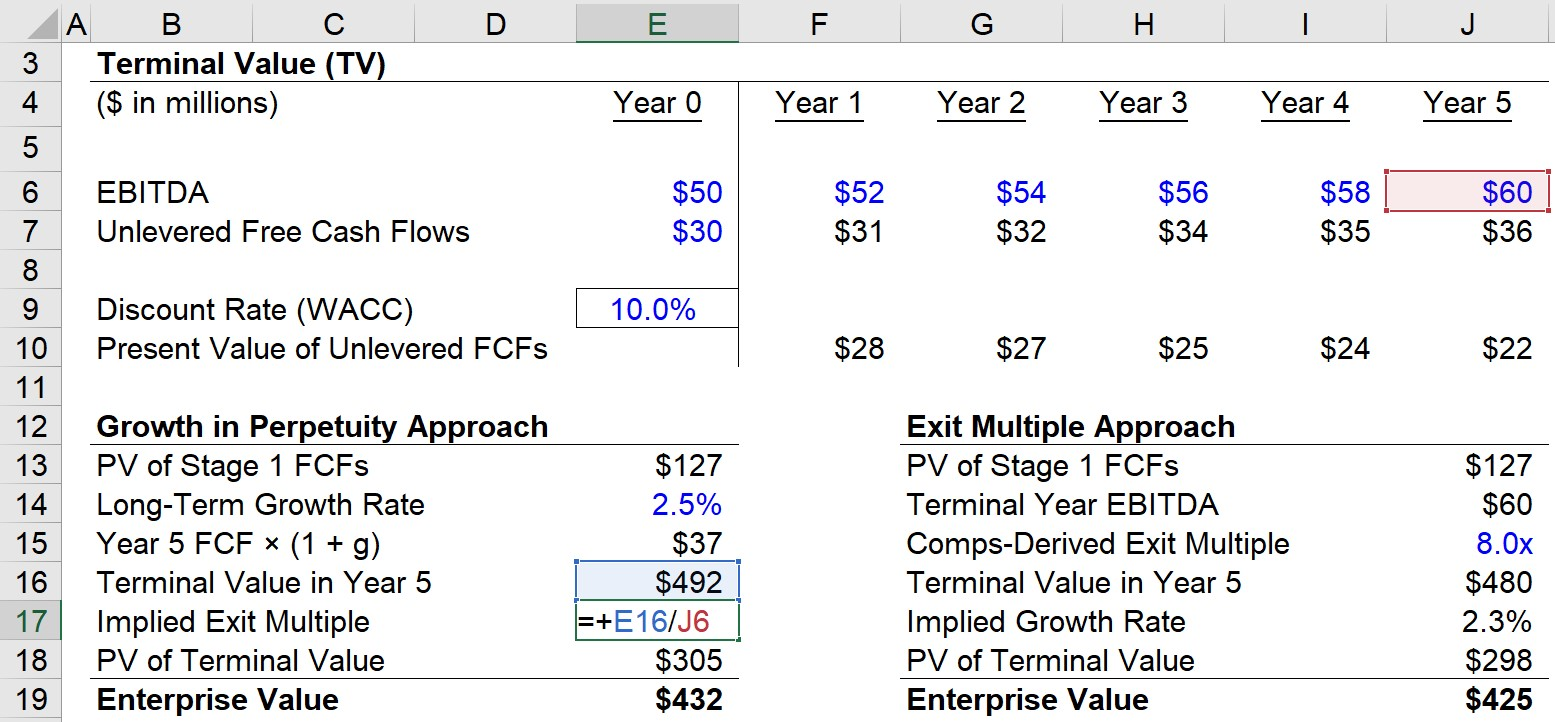In the subsequent step, we can now figure out the implied growth rate under the exit multiple approach. Considering the implied multiple from our perpetuity approach calculation based on a 2.5% long-term growth rate was 8.2x, the exit multiple assumption should be around that range. The exit multiple used was 8.0x, which comes out to a growth rate of 2.3% – a reasonable constant growth rate that confirms that our terminal value assumptions pass the sanity check.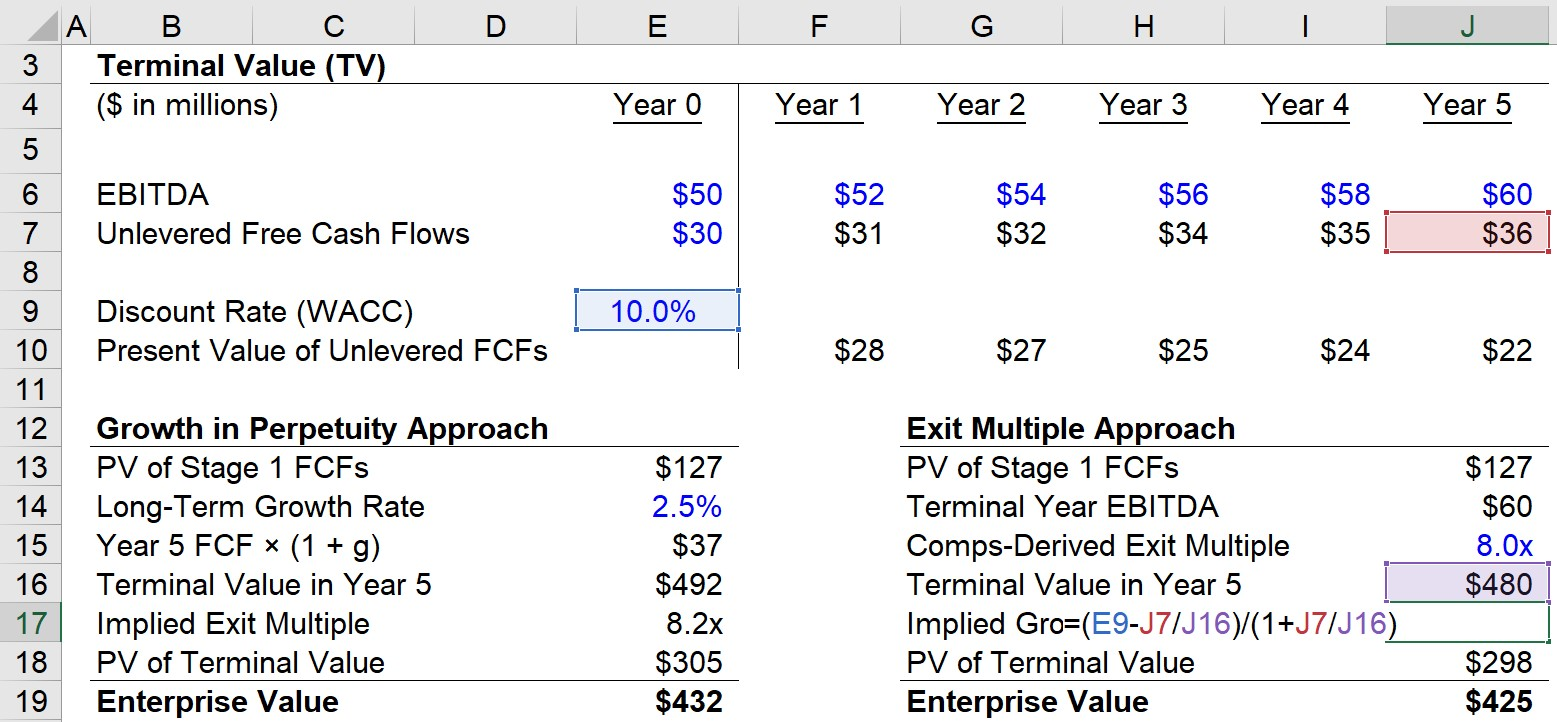Inline FeedbacksLearn Financial Modeling Online

Everything you need to master financial and valuation modeling: 3-Statement Modeling, DCF, Comps, M&A and LBO.

X

The Wall Street Prep Quicklesson Series

7 Free Financial Modeling Lessons

Get instant access to video lessons taught by experienced investment bankers. Learn financial statement modeling, DCF, M&A, LBO, Comps and Excel shortcuts.